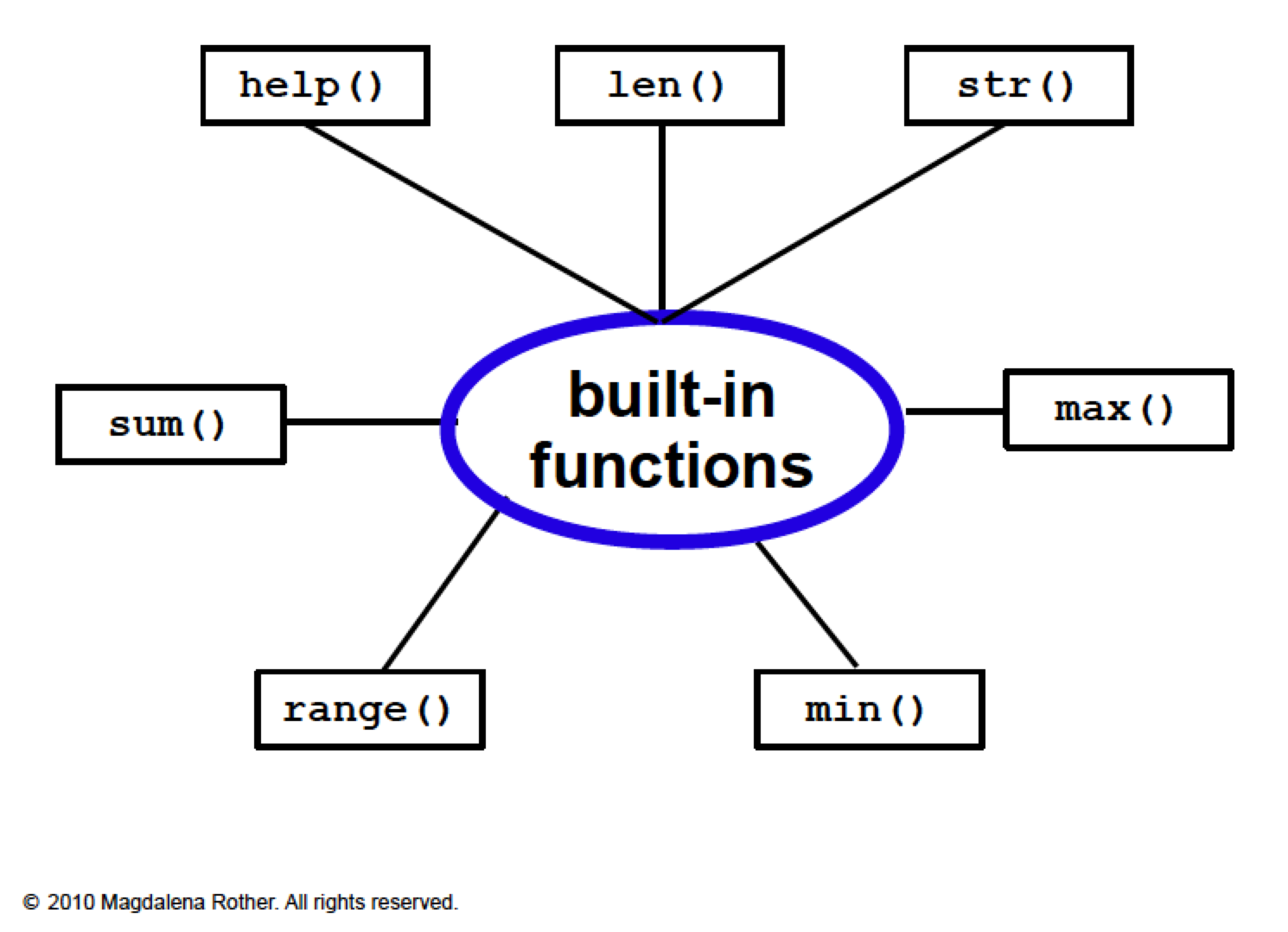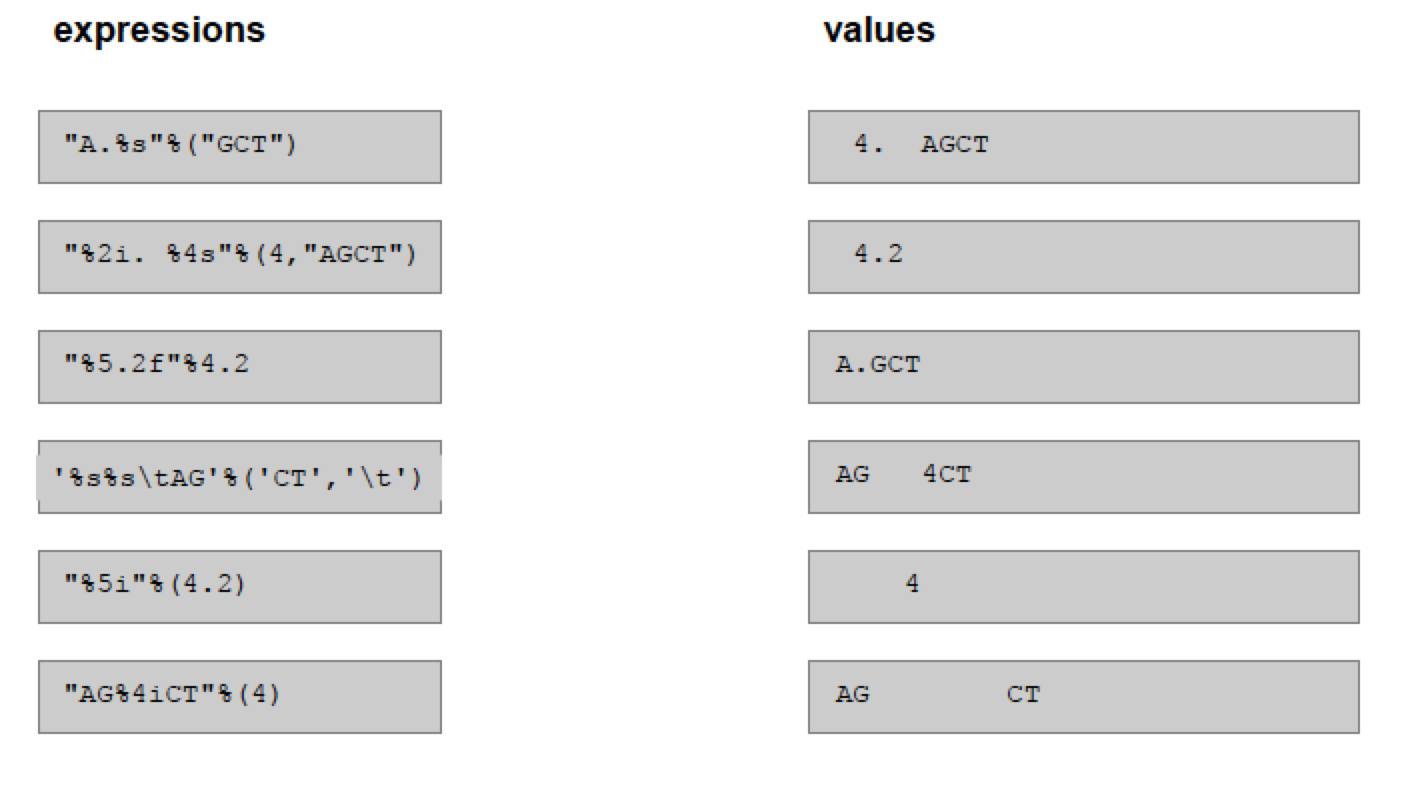## Reading data from a table and writing data to a table

After this module you can:

• Read a list of numbers from a text file to a list
• Calculate the average value of a list of numbers
• Calculate the average value of the numbers of a table’s column
• Write a list of numbers to a text fileWe will use the file `neuron_data.txt` that can be found here containing data on dendrites lengths:

``````16.38
139.90
441.46
29.03
40.93
202.07
142.30
346.00
300.00
``````

and the file `neuron_data-2.txt` available here that contains the same data plus an extra column with the indication 1 for primary neurons and 2 for secondary neurons:

``````1         16.38
2         139.90
2         441.46
1         29.03
1         40.93
2         202.07
1         142.30
2         346.00
2         300.00
``````

### What do we have to do?

• Read data columns from files
• Store data columns to data structures (lists)
• Convert text into numbers
• Convert numbers into text
• Write text to data columns (i.e. with appropriate format)

Our Goal is to beat Excel at its own game!

## Some useful built-in functions

• `split()` Stores elements from different columns to a list
• `unpack()` Stores elements from different columns to a list using a given format
• `join()` Concatenates objects from a list
• `strip()` Removes blank spaces and newline characters
• `int()` Converts a string into an integer
• `float()` Converts a string into a floating point number
• `str()` Converts an object into a string
• `repr()` Converts an object into a string

Challenge #1

Write a program that reads the file `neuron_data.txt` available here and stores neuron lengths as floating point numbers into a list.

See the Solution to challenge #1

Challenge #2

Extend the program so that it read data form `neuron_data-2.txt` available here and stores primary and secondary neuron lengths to different lists.

See the Solution to challenge #2

## Manipulating data in the columns

• Calculate max and min length
• Calculate their average length
• Calculate the standard deviationChallenge #3

Extend program 2 so that it calculates the neuron length average separately for primary and secondary neurons. Print the two averages: which neurons are on average longer?

See the Solution to challenge #3

Challenge #4

Extend program 3 so that it calculates the standard deviation of the neuron length.

See the Solution to challenge #4

## How to write data to columns

This implies that data needs to be nicely formatted and written to a file

• String concatenation
• String formatting

The argument of the `write()` function MUST be a string

``````>>> out = open('data_out.txt', 'w')
>>> out.write(3)
Traceback (most recent call last):
File "<stdin>", line 1, in <module>
TypeError: expected a character buffer object
>>>
``````
``````>>> out = open('data_out.txt', 'w')
>>> out.write(str(3))
>>>
``````

An example of string concatenation using `+` :

``````out = open('data_out.txt', 'w')
out.write(str(1) + '\t' + str(16.38) + '\n')
out.close()
``````

Challenge #5

Download the file `neuron_data-2.txt` from here. Use two lists with data from `neuron_data-2.txt` to write a table identical to `neuron_data-2.txt`. Do it using string concatenation.

See the Solution to challenge #5

## String formatting

Nice output can be generated by formatting characters:

``````print '%d'%(77)
print '%s'%('text')
print '%4.1f'%(2.1111)
``````
``````print 'The square root of %5.2f is %5.2f'%(a, math.sqrt(a))

print 'The square root of %10.2f is %5.2f'%(a, math.sqrt(a))

print "%i%s%f%s"%(1, '\t', 2.5, '\n')

import math
A = 25
S = 'The square root of {0} is  {1}'
print  S.format (a,  math.sqrt(a))
``````

Challenge #6

Use two lists with data from `neuron_data-2.txt` available here to write a table identical to `neuron_data-2.txt`. Do it using string formatting.

See the Solution to challenge #6

• Read each table column into a different list
• Use a for loop running over the length of the list to write the elements of the lists to a file (using string concatenation or formatting)
• You can write the columns in a different order

### Match the formatting expressions and their resultChallenge #7

Write a program that reads the file `neuron_data-2.txt` available here, calculates the number of primary neurons, their total length, and the shortest and the longest lengths.

Write the results to a file using string formatting.

You can repeat the exercise for secondary neurons.

See the Solution to challenge #7

### Back

Back to main page.